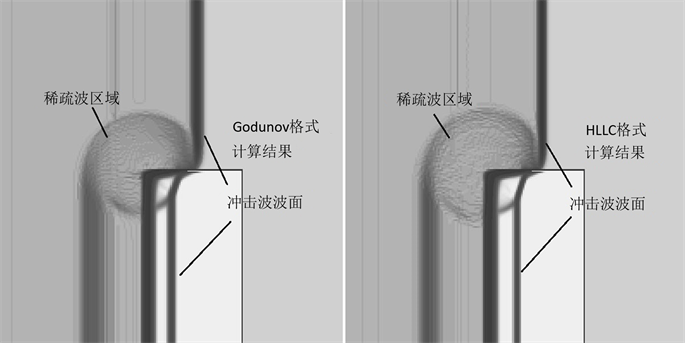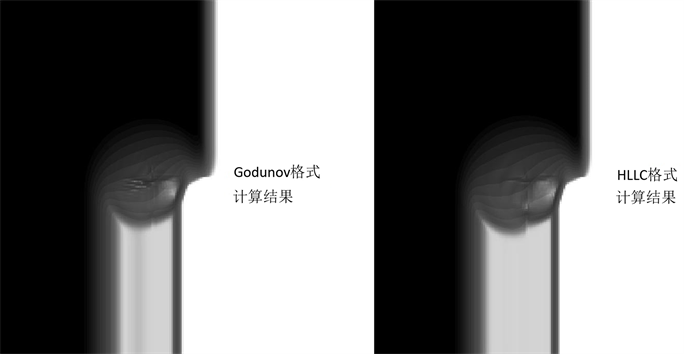# Mie-Grüneisen多介质混合计算模型的Godunov型格式The Godunov Type Scheme for Multi-Component Mie-Grüneisen Mixture Model

DOI: 10.12677/AAM.2019.82039, PDF, 下载: 341  浏览: 538  国家自然科学基金支持

Abstract: The aim of this article is the deduction of Godunov type scheme for Mie-Grüneisen mixture model. For multi-component problem with discontinuous sections, the Godunov schemes always keep the solution non-oscillation. To adapt to Mie-Grüneisen mixture model, the Godunov fluxes are recon-structed under finite volume method in 2D rectangular grids. According to numerical test, the Go-dunov scheme can couple well with Mie-Grüneisen mixture model.

1. 引言

2. 计算方法

2.1. 控制方程组

$\left\{\begin{array}{l}\frac{\partial \rho }{\partial t}+\frac{\partial }{\partial x}\left(\rho u\right)+\frac{\partial }{\partial y}\left(\rho v\right)=0\\ \frac{\partial \left(\rho u\right)}{\partial t}+\frac{\partial }{\partial x}\left(\rho {u}^{2}+p\right)+\frac{\partial }{\partial y}\left(\rho uv\right)=0\\ \frac{\partial \left(\rho v\right)}{\partial t}+\frac{\partial }{\partial x}\left(\rho uv\right)+\frac{\partial }{\partial y}\left(\rho {v}^{2}+p\right)=0\\ \frac{\partial \left(\rho E\right)}{\partial t}+\frac{\partial }{\partial x}\left(\rho Eu+pu\right)+\frac{\partial }{\partial y}\left(\rho Ev+pv\right)=0\end{array}$ (1)

$p-{p}_{ref}\left(\rho \right)=\rho \Gamma \left(\rho \right)\left(e-{e}_{ref}\left(\rho \right)\right)$ (2)

$\left\{\begin{array}{l}\frac{\partial }{\partial t}\left(\frac{1}{\Gamma }\right)+u\frac{\partial }{\partial x}\left(\frac{1}{\Gamma }\right)+v\frac{\partial }{\partial y}\left(\frac{1}{\Gamma }\right)+\rho \varphi \frac{\partial u}{\partial x}+\rho \varphi \frac{\partial v}{\partial y}=0\\ \frac{\partial }{\partial t}\left(\frac{{p}_{ref}}{\Gamma }\right)+u\frac{\partial }{\partial x}\left(\frac{{p}_{ref}}{\Gamma }\right)+v\frac{\partial }{\partial y}\left(\frac{{p}_{ref}}{\Gamma }\right)+\rho \phi \frac{\partial u}{\partial x}+\rho \phi \frac{\partial v}{\partial y}=0\\ \frac{\partial }{\partial t}\left(\rho {e}_{ref}\right)+u\frac{\partial }{\partial x}\left(\rho {e}_{ref}\right)+v\frac{\partial }{\partial y}\left(\rho {e}_{ref}\right)+\rho \psi \frac{\partial u}{\partial x}+\rho \psi \frac{\partial v}{\partial y}=0\end{array}$ (3)

$\frac{\partial \left(\rho {Y}_{\text{I}}\right)}{\partial t}+\frac{\partial \left(\rho {Y}_{\text{I}}u\right)}{\partial x}+\frac{\partial \left(\rho {Y}_{\text{I}}v\right)}{\partial y}=0,\text{\hspace{0.17em}}\text{\hspace{0.17em}}i=1,2,\cdot \cdot \cdot ,m-1$ (4)

2.2. 有限体积算法

Mie-Grüneisen多介质混合模型的有限体积格式可以表示成如下所示的形式：

$\frac{{W}_{j}^{n+1}-{W}_{j}^{n}}{\Delta t}+\frac{{F}_{i+1/2,j}-{F}_{i-1/2,j}}{\Delta x}+\frac{{G}_{i,j+1/2}-{G}_{i,j-1/2}}{\Delta y}=0$ (5)

$W=\left\{\begin{array}{c}\rho \\ \rho u\\ \rho v\\ \rho E\\ 1/\Gamma \\ {p}_{ref}/\Gamma \\ \rho {p}_{ref}\\ \rho {Y}_{\text{I}}\end{array}\right\}\text{ }$$F=\left\{\begin{array}{c}\rho u\\ \rho {u}^{2}+p\\ \rho uv\\ \left(\rho E+p\right)u\\ \rho \varphi u\\ \rho \phi u\\ \rho \psi u\\ \rho {Y}_{\text{I}}u\end{array}\right\}+u\left\{\begin{array}{c}0\\ 0\\ 0\\ 0\\ 1/\Gamma \\ {p}_{ref}/\Gamma \\ \rho {p}_{ref}\\ 0\end{array}\right\}$$G=\left\{\begin{array}{c}\rho u\\ \rho uv\\ \rho {v}^{2}+p\\ \left(\rho E+p\right)v\\ \rho \varphi v\\ \rho \phi v\\ \rho \psi v\\ \rho {Y}_{\text{I}}v\end{array}\right\}+v\left\{\begin{array}{c}0\\ 0\\ 0\\ 0\\ 1/\Gamma \\ {p}_{ref}/\Gamma \\ \rho {p}_{ref}\\ 0\end{array}\right\}$

Godunov型的通量F和G，其表达形式如下  ：

$\left\{\begin{array}{l}{F}_{i+1/2,j}=\frac{1}{2}\left[\text{ }{F}_{L}{\text{ }}_{i+1/2,j}+{F}_{R}{\text{ }}_{i+1/2,j}-\stackrel{^}{R}|\stackrel{^}{\Lambda }|\stackrel{^}{L}\left({W}_{L}{\text{ }}_{i+1/2,j}-{W}_{R}{\text{ }}_{i+1/2,j}\right)\right]\\ {G}_{i,j+1/2}=\frac{1}{2}\left[{G}_{L}{\text{ }}_{i,j+1/2}+{G}_{R}{\text{ }}_{i,j+1/2}-\stackrel{^}{R}|\stackrel{^}{\Lambda }|\stackrel{^}{L}\left({W}_{L}{\text{ }}_{i,j+1/2}-{W}_{R}{\text{ }}_{i,j+1/2}\right)\right]\end{array}$ (6)

2.3. 通量相关矩阵

${W}_{K}=\left\{\begin{array}{c}{\rho }_{K}\\ {\rho }_{K}{u}_{K}\\ {\rho }_{K}{v}_{K}\\ {\rho }_{K}{E}_{K}\\ {\left(1/\Gamma \right)}_{K}\\ {\left({p}_{ref}/\Gamma \right)}_{K}\\ {\left(\rho {p}_{ref}\right)}_{K}\\ {\left(\rho {Y}_{\text{I}}\right)}_{K}\end{array}\right\}\text{ }\text{ }$${F}_{K}或{G}_{K}=\left\{\begin{array}{c}{\left(\rho \theta \right)}_{K}\\ {\left(\rho \theta \right)}_{K}{u}_{K}+{p}_{K}{\zeta }_{x}\\ {\left(\rho \theta \right)}_{K}{v}_{K}+{p}_{K}{\zeta }_{y}\\ \left({\rho }_{K}{E}_{K}+{p}_{K}\right){\theta }_{K}\\ \theta {\left(1/\Gamma \right)}_{K}+\rho \varphi {\theta }_{K}\\ \theta {\left({p}_{ref}/\Gamma \right)}_{K}+\rho \phi {\theta }_{K}\\ \theta {\left(\rho {p}_{ref}\right)}_{K}+\rho \psi {\theta }_{K}\\ {\left(\rho {Y}_{I}\right)}_{K}{\theta }_{K}\end{array}\right\}\text{ }\text{ }\text{ }\text{ }\text{ }\text{ }\text{ }\text{ }\text{ }\text{ }\text{ }\text{ }\text{ }\text{ }\text{ }\text{ }\left(K=L,R\right)$ (7)

${\zeta }_{x}=\left\{\begin{array}{l}1\text{ }\text{ }\text{ }\text{ }\text{ }\text{ }\text{ }\text{ }\text{ }\text{ }\text{ }\text{ }\text{ }\text{ }\text{ }\text{ }\text{ }\text{ }\text{ }\text{ }通量F\text{ }\\ 0\text{ }\text{ }\text{ }\text{ }\text{ }\text{ }\text{ }\text{ }\text{ }\text{ }\text{ }\text{ }\text{ }\text{ }\text{ }\text{ }\text{ }\text{ }通量G\end{array},\text{ }\text{ }\text{ }\text{ }\text{ }\text{ }\text{ }\text{ }\text{ }\text{ }\text{ }\text{ }\text{ }\text{ }{\zeta }_{y}=\left\{\begin{array}{l}0\text{ }\text{ }\text{ }\text{ }\text{ }\text{ }\text{ }\text{ }\text{ }\text{ }\text{ }\text{ }\text{ }\text{ }\text{ }\text{ }\text{ }通量F\\ 1\text{ }\text{ }\text{ }\text{ }\text{ }\text{ }\text{ }\text{ }\text{ }\text{ }\text{ }\text{ }\text{ }\text{ }\text{ }\text{ }\text{ }\text{ }通量G\end{array}$

$A=\left[\begin{array}{cccccccc}& {\zeta }_{x}& \text{ }{\zeta }_{y}& & & & & \\ K{\zeta }_{x}-u\theta & \left(1-\Gamma \right)u{\zeta }_{x}+\theta & u{\zeta }_{y}-\Gamma v{\zeta }_{x}& \Gamma {\zeta }_{x}& -p\Gamma {\zeta }_{x}& \Gamma {\zeta }_{x}& -\Gamma {\zeta }_{x}& \\ K{\zeta }_{y}-v\theta & v{\zeta }_{x}-\Gamma u{\zeta }_{y}& \left(1-\Gamma \right)u{\zeta }_{y}+\theta & \Gamma {\zeta }_{y}& -p\Gamma {\zeta }_{y}& \Gamma {\zeta }_{y}& -\Gamma {\zeta }_{y}& \\ \left(K-H\right)\theta & H{\zeta }_{x}-\Gamma u\theta & H{\zeta }_{y}-\Gamma v\theta & \left(\Gamma +1\right)\theta & -p\Gamma \theta & \Gamma \text{ }\theta & -\text{ }\Gamma \theta & \\ -\varphi \theta & \varphi & & & \theta & & & \\ -\phi \theta & \phi & & & & \theta & & \\ -\psi \theta & \psi & & & & & \theta & \\ -{Y}_{\text{I}}\theta & {Y}_{\text{I}}& & & & & & \theta \end{array}\right]$ (8)

$\Lambda =\left[\begin{array}{cccccccc}\theta -c& & & & & & & \\ & \theta & & & & & & \\ & & \theta & & & & & \\ & & & \theta +c& & & & \\ & & & & \theta & & & \\ & & & & & \theta & & \\ & & & & & & \theta & \\ & & & & & & & \theta \end{array}\right]$

$c=\sqrt{\Gamma \left(H-\frac{1}{2}\left({u}^{2}+{v}^{2}\right)-p\varphi +\phi -\psi \right)}$ (9)

$L=\frac{\Gamma }{2{c}^{2}}\left[\begin{array}{cccccccc}\theta \xi +\frac{{u}^{2}+{v}^{2}}{2}& -u-{\zeta }_{x}\xi & -v-{\zeta }_{y}\xi & 1& -p& 1& -1& \\ -{u}^{2}-{v}^{2}+\kappa & 2u& 2v& -2& 2p& -2& 2& \\ \left({\zeta }_{y}u-{\zeta }_{x}v\right)\kappa & -{\zeta }_{y}\kappa & {\zeta }_{x}\kappa & & & & & \\ -\theta \xi +\frac{{u}^{2}+{v}^{2}}{2}& -u+{\zeta }_{x}\xi & -v+{\zeta }_{y}\xi & 1& -p& 1& -1& \\ -\varphi \left({u}^{2}+{v}^{2}\right)& 2\varphi u& 2\varphi v& -2\varphi & 2\varphi p+\kappa & -2\varphi & 2\varphi & \\ -\phi \left({u}^{2}+{v}^{2}\right)& 2\phi u& 2\phi v& -2\phi & 2\phi & -2\phi +\frac{\kappa }{\phi }& 2\phi & \\ -\psi \left({u}^{2}+{v}^{2}\right)& 2\psi u& 2\psi v& -2\psi & 2\psi & -2\psi & 2\psi +\frac{\kappa }{\psi }& \\ -Y\left({u}^{2}+{v}^{2}\right)& 2Yu& 2Yv& -2Y& 2Y& -2Y& 2Y& \kappa \end{array}\right]$

$R=\left[\begin{array}{cccccccc}1& 1& 0& 1& & & & \\ u-{\zeta }_{x}c& u& -{\zeta }_{y}& u+{\zeta }_{x}c& & & & \\ v-{\zeta }_{y}c& v& {\zeta }_{x}& v+{\zeta }_{y}c& & & & \\ H-\theta c& \frac{1}{2}\left({u}^{2}+{v}^{2}\right)& -{\zeta }_{y}u+{\zeta }_{x}v& H+\theta c& p& -1& 1& \\ \varphi & & & \varphi & 1& & & \\ \phi & & & \phi & & 1& & \\ \psi & & & \psi & & & 1& \\ Y& & & Y& & & & 1\end{array}\right]$

2.4. Roe平均值计算

$\stackrel{^}{u}=\frac{\sqrt{{\rho }_{L}}{u}_{L}+\sqrt{{\rho }_{R}}{u}_{R}}{\sqrt{{\rho }_{L}}+\sqrt{{\rho }_{R}}},\text{ }\text{ }\text{ }\text{ }\text{ }\text{ }\text{ }\text{ }\text{ }\text{ }\text{ }\stackrel{^}{v}=\frac{\sqrt{{\rho }_{L}}{v}_{L}+\sqrt{{\rho }_{R}}{v}_{R}}{\sqrt{{\rho }_{L}}+\sqrt{{\rho }_{R}}},\text{ }\text{ }\text{ }\text{ }\text{ }\text{ }\text{ }\text{ }\text{ }\text{ }\text{ }\text{ }\stackrel{^}{H}=\frac{\sqrt{{\rho }_{L}}{H}_{L}+\sqrt{{\rho }_{R}}{H}_{R}}{\sqrt{{\rho }_{L}}+\sqrt{{\rho }_{R}}}$

$\frac{1}{\stackrel{^}{\Gamma }}=\frac{\sqrt{{\rho }_{L}}\left(\text{ }1/{\Gamma }_{L}\right)+\sqrt{{\rho }_{R}}\left(1/{\Gamma }_{R}\right)}{\sqrt{{\rho }_{L}}+\sqrt{{\rho }_{R}}},\text{ }\text{ }\text{ }\text{ }\text{ }\text{ }\text{ }\text{ }\text{ }\frac{\stackrel{^}{p}}{\stackrel{^}{\Gamma }}=\frac{\sqrt{{\rho }_{L}}\left(\text{ }{p}_{L}/{\Gamma }_{L}\right)+\sqrt{{\rho }_{R}}\left(\text{ }{p}_{R}/{\Gamma }_{R}\right)}{\sqrt{{\rho }_{L}}+\sqrt{{\rho }_{R}}}$

3. 数值算例

$\Gamma ={\Gamma }_{0}{\left(\frac{V}{{V}_{0}}\right)}^{q},\text{ }\text{ }\text{ }\text{ }\text{ }\text{ }\text{ }\text{ }{p}_{ref}={p}_{0}+\frac{{c}_{0}{}^{2}\left({V}_{0}-V\right)}{{\left[{V}_{0}-s\left({V}_{0}-V\right)\right]}^{2}},\text{ }\text{ }\text{ }\text{ }\text{ }\text{ }\text{ }\text{ }\text{ }\text{ }\text{ }\text{ }{e}_{ref}={e}_{0}+\frac{1}{2}\left({p}_{ref}+{p}_{0}\right)\left({V}_{0}-V\right)$

$\begin{array}{l}\left\{\begin{array}{l}液态\text{MORB}:\rho =\text{ }\text{ }\text{ }\text{ }\text{ }\text{ }\text{ }\text{ }\text{ }2.26×{10}^{3}\text{\hspace{0.17em}}\text{kg}/{\text{m}}^{\text{3}},\text{ }\text{ }\text{ }\text{ }\text{ }\text{ }p=1×{10}^{5}\text{\hspace{0.17em}}\text{pa},\text{ }\text{ }\text{ }\text{ }\text{ }\text{ }\text{ }\text{ }\text{ }\text{ }\text{ }\text{ }\text{ }u=0,\\ 正常态钼:\rho =\text{ }\text{ }\text{ }\text{ }\text{ }\text{ }\text{ }\text{ }\text{ }\text{ }9.961×{10}^{3}\text{\hspace{0.17em}}\text{kg}/{\text{m}}^{\text{3}},\text{ }\text{ }\text{ }\text{ }\text{ }\text{ }p=1×{10}^{5}\text{\hspace{0.17em}}\text{pa},\text{ }\text{ }\text{ }\text{ }\text{ }\text{ }\text{ }\text{ }\text{ }\text{ }\text{ }\text{ }\text{ }u=0\\ 压缩态钼:\rho =11.042×{10}^{3}\text{\hspace{0.17em}}\text{kg}/{\text{m}}^{\text{3}},\text{ }\text{ }\text{ }\text{ }\text{ }\text{ }\text{ }p=3×{10}^{10}\text{\hspace{0.17em}}\text{pa},\text{ }\text{ }\text{ }\text{ }\text{ }\text{ }\text{ }\text{ }\text{ }u=543\text{\hspace{0.17em}}\text{m}/\text{s}\end{array}\\ 液态\text{MORB}:{\rho }_{0}=2.26×{10}^{3}\text{\hspace{0.17em}}\text{kg}/{\text{m}}^{\text{3}},\text{ }\text{ }\text{ }\text{ }\text{ }{c}_{0}=2100\text{\hspace{0.17em}}\text{m}/\text{s},\text{ }\text{ }\text{ }\text{ }s=1.68,\text{ }\text{ }\text{ }\text{ }{\Gamma }_{0}=1.56,\text{ }\text{ }\text{ }q=1.0\\ 钼:{\rho }_{0}=9.96×{10}^{3}\text{\hspace{0.17em}}\text{kg}/{\text{m}}^{\text{3}},\text{ }\text{ }\text{ }\text{ }\text{ }{c}_{0}=4770\text{\hspace{0.17em}}\text{m}/\text{s}\text{ },\text{ }\text{ }\text{ }\text{ }s=1.43,\text{ }\text{ }\text{ }\text{ }{\Gamma }_{0}=0.18,\text{ }\text{ }\text{ }q=1.0\end{array}$Figure 1. Density contours of Godunov scheme (left) and HLLC scheme (right)Figure 2. Pressure contours of Godunov scheme (left) and HLLC scheme (right)

4. 结论

  Toro, E.F. (2009) Riemann Solvers and Numerical Methods for Fluid Dynamics. Springer-Verlag, Berlin Heidel-berg.  王东红, 赵宁, 徐爽. 适用于Mie-Grüneisen状态方程的一种通用激波限制器[J]. 南京航空航天大学学报, 2014, 46(2): 232-238.  李晓杰, 闫鸿浩, 王小红, 等. Mie-Grüneisen状态方程的物理力学释义[J]. 高压物理学报, 2014, 28(2): 227-231.  Shyue, K.-M. (2001) A Fluid-Mixture Type Algorithm for Compressible Mul-ticomponent Flow with Mie-Grüneisen Equation of States. Journal of Computational Physics, 171, 678-707. https://doi.org/10.1006/jcph.2001.6801  吴宗铎, 宗智. Mie-Grüneisen状态方程下多介质守恒型欧拉方程组的数值计算[J]. 计算物理, 2011, 28(6): 803-809.  吴宗铎, 严谨, 宗智, 等. 一种基于HLLC算法的Mie-Grüneisen多介质混合模型[EB/OL]. 计算物理. http://kns.cnki.net/kcms/detail/11.2011.o4.20181219.1145.004.html, 2018-12-24.  任玉新, 陈海昕. 计算流体力学基础[M]. 北京: 清华大学出版社, 2006.  刘晓慧, 李肖. ALE框架下虚拟流体方法模拟移动边界的一维可压缩多介质流动问题[J]. 应用数学进展, 2018, 7(12): 1638-1644.